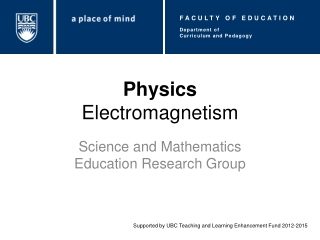DownloadDownload PresentationPhysics Electromagnetism

# Physics Electromagnetism

Download Presentation## Physics Electromagnetism

- - - - - - - - - - - - - - - - - - - - - - - - - - - E N D - - - - - - - - - - - - - - - - - - - - - - - - - - -
##### Presentation Transcript

1. FACULTY OF EDUCATION Department of Curriculum and Pedagogy PhysicsElectromagnetism Science and Mathematics Education Research Group Supported by UBC Teaching and Learning Enhancement Fund 2012-2015

2. Electromagnetism Question Title Question Title http://www.hese-project.org/hese-uk/en/niemr/

3. Electromagnetism Question Title Question Title The following questions have been compiled from a collection of questions submitted on PeerWise (https://peerwise.cs.auckland.ac.nz/) by teacher candidates as part of the EDCP 357 physics methods courses at UBC.

4. Electromagnetism Problems I Question Title Question Title The figures below show straight wire segments carrying current in uniform magnetic fields (i.e. same strength). Can you rank these situations from the greatest to the least on the basis of the strength (magnitude) of the magnetic force on each wire. If the situations have the same strength, then rank them next alphabetically. Note that: X – represents magnetic fields directed into the screen. X – represents magnetic fields coming out of the screen.

5. Electromagnetism Problems I (cont.) Question Title Question Title A. D, B, E, A, C, F B. A, C, F, D, B, E C. D, A, E, F, C, B D. Same force in all six cases. E. Impossible to rank the forces.

6. Solution Question Title Question Title Answer:D Justification:Remember, the force on a sectionof wire of length carrying a current through amagnetic field is given by ,where is the angle between the wire and themagnetic field. In figures A, C, and F, . In figure B, . In figures D and E, . In all the cases, the strength of the magnetic force on each wire is the same, that is . Therefore, D is the correct answer.

7. Electromagnetism Problems II Question Title Question Title The figures below show straight wire segments carrying current in uniform magnetic fields (i.e. same strength) directed into the screen. Can you rank these situations from the greatest to the least on the basis of the strength (magnitude) of the magnetic force on each wire. If the situations have the same strength, then rank them next alphabetically.

8. Electromagnetism Problems II (cont.) Question Title Question Title A. A, B, C, D, E, F B. C, D, A, B, E, F C. E, F, C, D, A, B D. Same force in all six cases. E. Impossible to rank the forces.

9. Solution Question Title Question Title Answer: B Justification:Remember, the force on a section of wire of length carrying a current through a magnetic field is given by , where is the angle between the wire and themagnetic field. Note that in all the cases. Since , and are constant, we know that is proportional to , that is . In figures A, B, E, and F, . In figures C and D, . Thus, . Therefore, B is the correct answer. Watch: https://www.youtube.com/watch?v=nfSJ62mzKyY

10. Electromagnetism Problems III Question Title Question Title Consider the following solenoid circuit with a DC power supply. Given the magnetic field lines, which diagram best represents the induced magnetization of the metal bar within the coil of wire? Options are presented in the next slide. Power Supply

11. Electromagnetism Problems III (cont.) Question Title Question Title A B North South North South South North North South C D Power Supply Power Supply Power Supply Power Supply

12. Solution Question Title Question Title Answer:D Justification: Note that we do not need to know the direction of the current. By convention, magnetic field lines emerge from the North pole of a magnet and enter at the South pole. Considering the magnetic field line, we see that the field lines are emerging from the left side of the solenoid and entering at the right side of the solenoid. Therefore, D is the correct answer. Watch: https://www.youtube.com/watch?v=V-M07N4a6-Y